# The ROBUSTREG Procedure

### PROC ROBUSTREG Statement

Subsections:
• PROC ROBUSTREG <options>;

The PROC ROBUSTREG statement invokes the ROBUSTREG procedure. Table 98.1 summarizes the options available in the PROC ROBUSTREG statement.

Table 98.1: PROC ROBUSTREG Statement Options

Option

Description

Saves the estimated covariance matrix

Specifies the input SAS data set

Computes the final weighted least squares estimates

Specifies an input SAS data set that contains initial estimates

Displays the iteration history of the iteratively reweighted least squares algorithm

Specifies the estimation method

Specifies the length of effect names

Specifies the order in which to sort classification variables

Specifies an output SAS data set that contains the parameter estimates

Specifies options that control details of the plots

Specifies the seed for the random number generator

You can specify the following options in the PROC ROBUSTREG statement.

COVOUT

saves the estimated covariance matrix in the OUTEST= data set. This option is not supported for LTS estimation.

DATA=SAS-data-set

specifies the input SAS data set to be used by PROC ROBUSTREG. By default, the most recently created SAS data set is used.

FWLS

computes the final weighted least squares estimates. These estimates are equivalent to the least squares estimates after the detected outliers are deleted.

INEST=SAS-data-set

specifies an input SAS data set that contains initial estimates for all the parameters in the model. For a detailed description of the contents of the INEST= data set, see the section INEST= Data Set.

ITPRINT

displays the iteration history of the iteratively reweighted least squares algorithm that is used in M and MM estimation. You can also use this option in the MODEL statement.

METHOD=method-type <(options)>

specifies the estimation method and some additional options for the estimation method. PROC ROBUSTREG provides four estimation methods: M estimation, LTS estimation, S estimation, and MM estimation. The default method is M estimation.

Note: Because the LTS and S methods use subsampling algorithms, these methods are not suitable in an analysis that uses variables that have only a few unequal values or a few unequal values within one BY group. For example, indicator variables that correspond to a classification variable often fall into this category. The same issue also applies to the initial LTS and S estimates in the MM method. For a model that includes classification independent variables or continuous independent variables with a few unequal values, the M method is recommended.

NAMELEN=n

specifies the length of effect names in tables and output data sets to be n characters, where n is a value between 20 and 200. The default length is 20 characters.

ORDER=DATA | FORMATTED | FREQ | INTERNAL

specifies the sort order for the levels of the classification variables (which are specified in the CLASS statement).

This option applies to the levels for all classification variables, except when you use the (default) ORDER=FORMATTED option with numeric classification variables that have no explicit format. In that case, the levels of such variables are ordered by their internal value.

The ORDER= option can take the following values:

Value of ORDER=

Levels Sorted By

DATA

Order of appearance in the input data set

FORMATTED

External formatted value, except for numeric variables with no explicit format, which are sorted by their unformatted (internal) value

FREQ

Descending frequency count; levels with the most observations come first in the order

INTERNAL

Unformatted value

By default, ORDER=FORMATTED. For ORDER=FORMATTED and ORDER=INTERNAL, the sort order is machine-dependent.

For more information about sort order, see the chapter on the SORT procedure in the Base SAS Procedures Guide and the discussion of BY-group processing in SAS Language Reference: Concepts.

OUTEST=SAS-data-set

specifies an output SAS data set that contains the parameter estimates and, if the COVOUT option is specified, the estimated covariance matrix. For a detailed description of the contents of the OUTEST= data set, see the section OUTEST= Data Set.

PLOT | PLOTS <(global-plot-options)> <=plot-request>
PLOT | PLOTS <(global-plot-options)> <=(plot-request < …plot-request > )>

specifies options that control details of the plots. If ODS Graphics is enabled but you do not specify the PLOTS= option, then PROC ROBUSTREG produces the robust fit plot by default when the model includes a single continuous independent variable.

ODS Graphics must be enabled before plots can be requested. For example:

ods graphics on;
proc robustreg data=stack plots=all;
model y = x1 x2 x3;
run;
ods graphics off;


For more information about enabling and disabling ODS Graphics, see the section Enabling and Disabling ODS Graphics in Chapter 21: Statistical Graphics Using ODS.

The global-plot-options apply to all plots that are generated by the ROBUSTREG procedure. The following global-plot-option is available:

ONLY

suppresses the default robust fit plot. Only plots that are specifically requested are displayed.

You can specify more than one plot-request within the parentheses after PLOTS=. For a single plot-request, you can omit the parentheses. The following plot-requests are available:

ALL

creates all appropriate plots.

DDPLOT <(LABEL=ALL | LEVERAGE | NONE | OUTLIER)>

creates a plot of robust distance against Mahalanobis distance. For more information about robust distance, see the section Leverage-Point and Outlier Detection. The LABEL= option specifies how the points in this plot are to be labeled, as summarized in Table 98.2.

Table 98.2: Options for Label

Value of LABEL=

Label Method

ALL

Label all points

LEVERAGE

Label leverage points

NONE

No labels

OUTLIERS

Label outliers

By default, the ROBUSTREG procedure labels both outliers and leverage points.

If you specify ID variables in the ID statement, the values of the first ID variable are used as labels; otherwise, observation numbers are used as labels.

FITPLOT <(NOLIMITS)>

creates a plot of robust fit against the single independent continuous variable that is specified in the model. You can request this plot when only a single independent continuous variable is specified in the model. Confidence limits are added to the plot by default. The NOLIMITS option suppresses these limits.

HISTOGRAM

creates a histogram for the standardized robust residuals. The histogram is superimposed with a normal density curve and a kernel density curve.

NONE

suppresses all plots.

QQPLOT

creates the normal quantile-quantile plot for the standardized robust residuals.

RDPLOT <(LABEL=ALL | LEVERAGE | NONE | OUTLIER)>

creates the plot of standardized robust residual against robust distance. For more information about robust distance, see the section Leverage-Point and Outlier Detection. The LABEL= option specifies a label method for points in this plot. These label methods are described in Table 98.2.

If you specify ID variables in the ID statement, the values of the first ID variable are used as labels; otherwise, observation numbers are used as labels.

SEED=number

specifies the seed for the random number generator used to randomly select the subgroups and subsets for LTS and S estimation. By default, or if you specify 0, the ROBUSTREG procedure generates a random seed.

#### Options with METHOD=M

When you specify METHOD=M <(options)>, you can specify the following options:

ASYMPCOV=H1 | H2 | H3

specifies the type of asymptotic covariance that is computed for the M estimate. The three types are described in the section Asymptotic Covariance and Confidence Intervals. By default, ASYMPCOV=H1.

CONVERGENCE=criterion <(EPS=value)>

specifies a convergence criterion for the M estimate. Table 98.3 lists the three criteria that are available.

Table 98.3: Options to Specify Convergence Criteria

Type

Option

Coefficient

CONVERGENCE=COEF

Residual

CONVERGENCE=RESID

Weight

CONVERGENCE=WEIGHT

By default, CONVERGENCE=COEF. You can specify the precision of the convergence criterion by using the EPS= option; by default, EPS=1E–8.

MAXITER=n

sets the maximum number of iterations during the parameter estimation. By default, MAXITER=1000.

SCALE=scale-type | value

specifies the scale parameter or a method of estimating the scale parameter. These methods and options are summarized in Table 98.4.

Table 98.4: Options to Specify Scale

Scale

Option

Default d

Fixed constant

SCALE=value

Huber estimate

SCALE=HUBER <(D=d)>

2.5

Median estimate

SCALE=MED

Tukey estimate

SCALE=TUKEY <(D=d)>

2.5

By default, SCALE=MED.

WEIGHTFUNCTION=function-type
WF=function-type

specifies the weight function that is used for the M estimate. The ROBUSTREG procedure provides 10 weight functions, which are listed in Table 98.5. You can specify the parameters in these functions by using the A=, B=, and C= options. These functions are described in the section M Estimation. The default weight function is bisquare.

Table 98.5: Options to Specify Weight Functions

Weight Function

Option

Default a, b, c

Andrews

WF=ANDREWS <(C=c)>

1.339

Bisquare

WF=BISQUARE <(C=c)>

4.685

Cauchy

WF=CAUCHY <(C=c)>

2.385

Fair

WF=FAIR <(C=c)>

1.4

Hampel

WF=HAMPEL <(<A=a> <B=b> <C=c>)>

2, 4, 8

Huber

WF=HUBER <(C=c)>

1.345

Logistic

WF=LOGISTIC <(C=c)>

1.205

Median

WF=MEDIAN <(C=c)>

0.01

Talworth

WF=TALWORTH <(C=c)>

2.795

Welsch

WF=WELSCH <(C=c)>

2.985

#### Options with METHOD=LTS

When you specify METHOD=LTS <(options)>, you can specify the following options:

CSTEP=n

specifies the number of concentration steps (C-steps) for the LTS estimate. For information about how the default value is determined, see the section LTS Estimate.

H=n

specifies the quantile for the LTS estimate. For information about how the default value is determined, see the section LTS Estimate

requests (IADJUST=ALL) or suppresses (IADJUST=NONE) the intercept adjustment for all estimates in the LTS algorithm. By default, the intercept adjustment is used for data sets that contain fewer than 10,000 observations. For more information, see the section Algorithm.

NBEST=n

specifies the number of best solutions that are kept for each subgroup during the computation of the LTS estimate. The default number is 10, which is the maximum number allowed.

NREP=n

specifies the number of times to repeat least squares fit in subgroups during the computation of the LTS estimate. For information about how the default number is determined, see the section LTS Estimate.

SUBANALYSIS

requests a display of the subgrouping information and parameter estimates within subgroups. This option generates the ODS tables that are listed in Table 98.6.

Table 98.6: ODS Tables Available with SUBANALYSIS Option

ODS Table Name

Description

BestEstimates

Best final estimates for LTS

BestSubEstimates

Best estimates for each subgroup

CStep

C-step information for LTS

Groups

Grouping information for LTS

SUBGROUPSIZE=n

specifies the data set size of the subgroups in the computation of the LTS estimate. The default number is 300.

#### Options with METHOD=S

When you specify METHOD=S <(options)>, you can specify the following options:

ASYMPCOV=H1 | H2 | H3 | H4

specifies the type of asymptotic covariance that is computed for the S estimate. The four types are described in the section Asymptotic Covariance and Confidence Intervals. By default, ASYMPCOV=H4.

CHIF= TUKEY | YOHAI

specifies the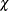function for the S estimate. PROC ROBUSTREG provides twofunctions, Tukey’s bisquare function and Yohai’s optimal function, which you can request by specifying CHIF=TUKEY and CHIF=YOHAI, respectively. The default is Tukey’s bisquare function.

EFF=value

specifies the efficiency (as a fraction) of the S estimate. The parameter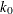in thefunction is determined by this efficiency. The default efficiency is determined such that the consistent S estimate has a breakdown value of 25%. This option is overwritten by the K0= option if both options are used.

K0=value

specifies theparameter in thefunction of the S estimate. If you specify CHIF=TUKEY, the default is 1.548. If you specify CHIF=YOHAI, the default is 0.66. These default values correspond to a 50% breakdown value of the consistent S estimate.

MAXITER=n

sets the maximum number of iterations for computing the scale parameter of the S estimate. By default, MAXITER=1000.

NREP=n

specifies the number of repeats of subsampling in the computation of the S estimate. For information about how the default number of repeats is determined, see the section Algorithm.

NOREFINE

suppresses the refinement of the S estimate. For more information, see the section Algorithm.

SUBSETSIZE=n

specifies the size of the subset for the S estimate. For information about how the default value is determined, see the section Algorithm.

TOLERANCE=value

specifies the tolerance for the S estimate of the scale. The default value is 0.001.

#### Options with METHOD=MM

When you specify METHOD=MM <(options)>, you can specify the following options:

ASYMPCOV=H1 | H2 | H3 | H4

specifies the type of asymptotic covariance that is computed for the MM estimate. The four types are described in the section Details: ROBUSTREG Procedure. By default, ASYMPCOV=H4.

BIASTEST <(ALPHA=number)>

requests the bias test for the final MM estimate. For more information about this test, see the section Bias Test.

CHIF=TUKEY | YOHAI

selects thefunction for the MM estimate. PROC ROBUSTREG provides twofunctions, Tukey’s bisquare function and Yohai’s optimal function, which you can request by specifying CHIF=TUKEY and CHIF=YOHAI, respectively. The default is Tukey’s bisquare function. Thisfunction is also used by the initial S estimate if you specify the INITEST=S option.

CONVERGENCE=criterion <(EPS=number)>

specifies a convergence criterion for the MM estimate. Table 98.7 lists the three criteria that are available.

Table 98.7: Options to Specify Convergence Criteria

Type

Option

Coefficient

CONVERGENCE=COEF

Residual

CONVERGENCE=RESID

Weight

CONVERGENCE=WEIGHT

By default, CONVERGENCE=COEF. You can specify the precision of the convergence criterion by using the EPS= option; by default, EPS=1E–8.

EFF=value

specifies the efficiency (as a fraction) of the MM estimate. The parameter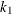in thefunction is determined by this efficiency. The default efficiency is set to 0.85, which corresponds to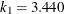if you specify CHIF=TUKEY or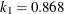if you specify CHIF=YOHAI.

INITEST=LTS | S

specifies the initial estimator for the MM estimator. By default, the LTS estimator with its default settings is used as the initial estimator for the MM estimator.

INITH=h

specifies the integer h for the initial LTS estimate that is used by the MM estimator. For information about how to specify h and how the default is determined, see the section Algorithm.

K0=number

specifies the parameterin thefunction for the MM estimate. If you specify CHIF=TUKEY, the default is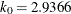. If you specify CHIF=YOHAI, the default is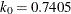. These default values correspond to the 25% breakdown value of the MM estimator.

MAXITER=n

sets the maximum number of iterations during the parameter estimation. By default, MAXITER=1000.